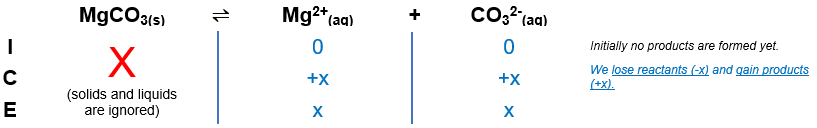# Problem: Determine the molar solubility of MgCO3 in pure water. Ksp (MgCO3) = 6.82x10-6

###### FREE Expert Solution

MgCO3(s)  Mg2+(aq) + CO32(aq)The Ksp expression for MgCO3 is:

$\overline{){{\mathbf{K}}}_{{\mathbf{sp}}}{\mathbf{=}}\frac{\mathbf{products}}{\overline{)\mathbf{reactants}}}{\mathbf{=}}{\mathbf{\left[}}{{\mathbf{Mg}}}^{\mathbf{2}\mathbf{+}}{\mathbf{\right]}}{\mathbf{\left[}}{{{\mathbf{CO}}}_{{\mathbf{3}}}}^{{\mathbf{2}}{\mathbf{-}}}{\mathbf{\right]}}}$

Calculate molar solubility of MgCO3 in M or mol/L (x):

93% (132 ratings)###### Problem Details

Determine the molar solubility of MgCOin pure water. Ksp (MgCO3) = 6.82x10-6